# Solution assignment 04 Exponential functions and graphs

### Assignment 4

Draw the graph of the function: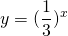What is the formula of the asymptote?

### Solution

Here the base is less thanand therefore the graph is the mirror of the graph of the function: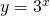We can show this quite easily. We write: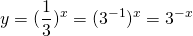This means that the graph is the mirror in the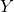-axis of the graph of: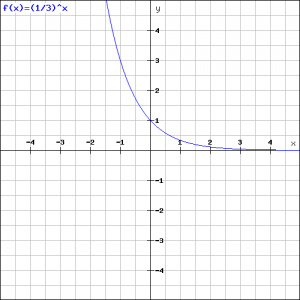The asymptote is the-axis, i.e.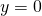, because the larger the value ofthe more the graph approaches the-axis. We can verify this in the figure.

0